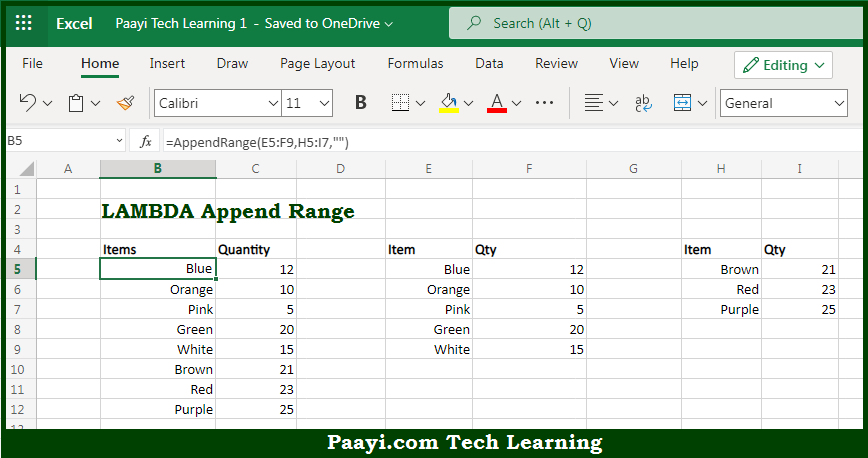# Learn How to Get LAMBDA Append Range in Microsoft Excel

Written by | 0 Comments | 475 Views

In this article, you will learn how to evaluate things in Dynamic Arrays with formulas in Microsoft Excel using a single/combination(s) of functions. You will also know How to Get LAMBDA Append Range and see the generic formula.

Learn How to Get LAMBDA Append Range in Microsoft Excel

The main purpose of this formula is to get LAMBDA to append range. Here we will learn how to get LAMBDA to append range in the workbook in Microsoft Excel. That implies, with the help of a formula based on the LAMBDA function you can able to get appended LAMBDA range. So, with the help of this formula, you can able to get LAMBDA to append range in the workbook in Microsoft Excel.

General Formula to LAMBDA Append Range

=AppendRange(range1,range2,default)

The Explanation for LAMBDA Append RangeSo we know that with the help of the given formula above you can able to get LAMBDA to append range. Here we will learn how to get LAMBDA to append range in the workbook in Microsoft Excel. As we know that Excel does not provide a formula function to append or combine ranges. So you can append ranges with Power Query. You can also use the LAMBDA function to create a custom function to combine ranges. So, with the help of this formula, you can able to get LAMBDA to append range. Here we will learn how to get LAMBDA to append range in the workbook in Microsoft Excel.